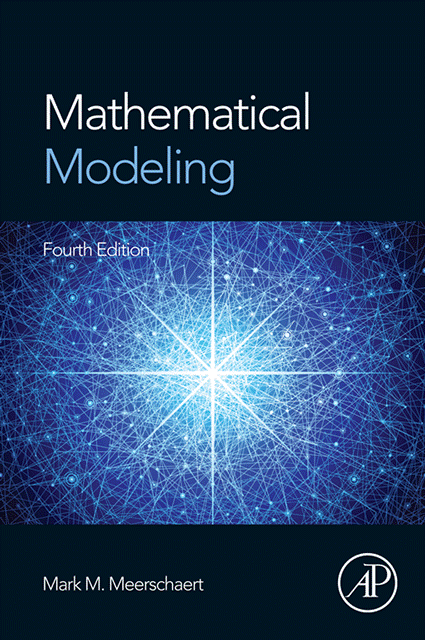Mathematical Modeling by Mark M. Meerschaert
The fourth edition of the text (Academic Press, Elsevier, ISBN: 978-0-12-386912-8) is now available.  See updated table of contents below. New sections on particle tracking and anomalous diffusion (including fractional calculus) are included in the fourth edition of the text.  To request a review copy of the text, or to get a copy of the instructor's solutions manual, visit the Elsevier web site at textbooks.elsevier.com or send email to textbook@elsevier.com.   Click here for errata to the 4th edition.

The text is intended to serve as a general introduction to the area of mathematical modeling, aimed at advanced undergraduate or beginning graduate students in mathematics and closely related fields. Formal prerequisites consist of the usual freshman-sophomore sequence in mathematics, including one-variable calculus, multivariable calculus, linear algebra, and differential equations. Prior exposure to computing and probability and statistics is useful, but is not required.

Unlike some textbooks that focus on one kind of mathematical model, this book covers the broad spectrum of modeling problems, from optimization to dynamical systems to stochastic processes. Each chapter in this book is followed by a set of challenging exercises. Following the exercises in each chapter is a list of suggestions for further reading. This list includes references to a number of UMAP modules in applied mathematics which are relevant to the material in the chapter. UMAP modules can provide interesting supplements to the material in the text, or extra credit projects. All of the UMAP modules are available at a nominal cost from COMAP, Inc., Suite 210, 57 Bedford Street, Lexington MA 02137.

The text contains numerous computer-generated graphs, along with instruction on the appropriate use of graphing utilities in mathematics. Computer algebra systems are used extensively in those chapters where significant algebraic calculation is required. The text includes actual computer output from the computer algebra systems MAPLE and MATHEMATICA, the popular linear programming package LINDO, and the commonly used statistical package MINITAB.

• I. OPTIMIZATION MODELS
• 1. One-Variable Optimization
• 1.1 The Five-Step Method
• 1.2 Sensitivity Analysis
• 1.3 Sensitivity and Robustness
• 2. Multivariable Optimization
• 2.1 Unconstrained Optimization
• 2.2 Lagrange Multipliers
• 2.3 Sensitivity Analysis and Shadow Prices
• 3. Computational Methods for Optimization
• 3.1 One-Variable Optimization
• 3.2 Multivariable Optimization
• 3.3 Linear Programming
• 3.4 Discrete Optimization
• II. DYNAMIC MODELS
• 4. Introduction to Dynamic Models
• 4.2 Dynamical Systems
• 4.3 Discrete-Time Dynamical Systems
• 5. Analysis of Dynamic Models
• 5.1 Eigenvalue Methods
• 5.2 Eigenvalue Methods for Discrete Systems
• 5.3 Phase Portraits
• 6. Simulation of Dynamic Models
• 6.1 Introduction to Simulation
• 6.2 Continuous-Time Models
• 6.3 The Euler Method
• 6.4 Chaos and Fractals
• III. PROBABILITY MODELS
• 7. Introduction to Probability Models
• 7.1 Discrete Probability Models
• 7.2 Continuous Probability Models
• 7.3 Introduction to Statistics
• 7.4 Diffusion
• 8. Stochastic Models
• 8.1 Markov Chains
• 8.2 Markov Processes
• 8.3 Linear Regression
• 8.4 Time Series
• 9. Simulation of Probability Models
• 9.1 Monte Carlo Simulation
• 9.2 The Markov Property
• 9.3 Analytic Simulation
• 9.4 Particle Tracking (new for 4/ed)
• 9.5 Anomalous Diffusion (new for 4/ed)